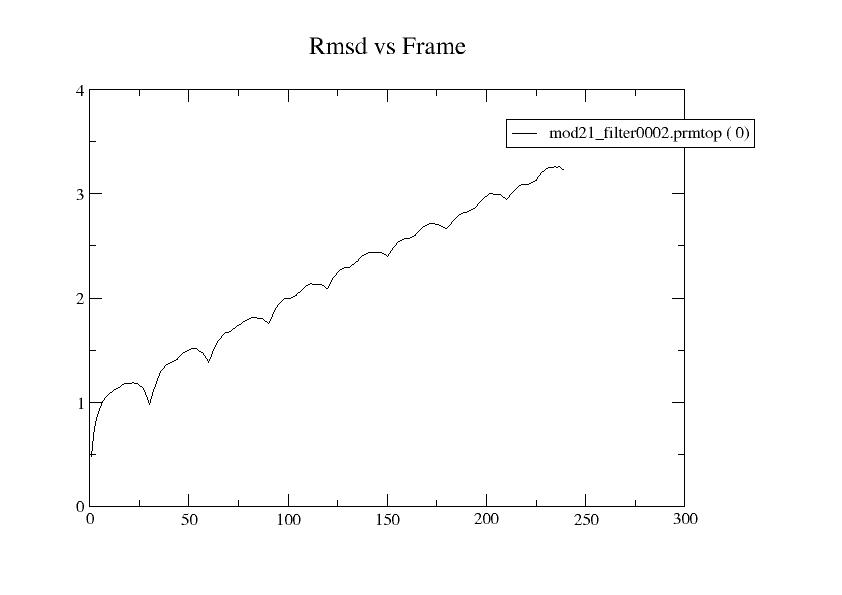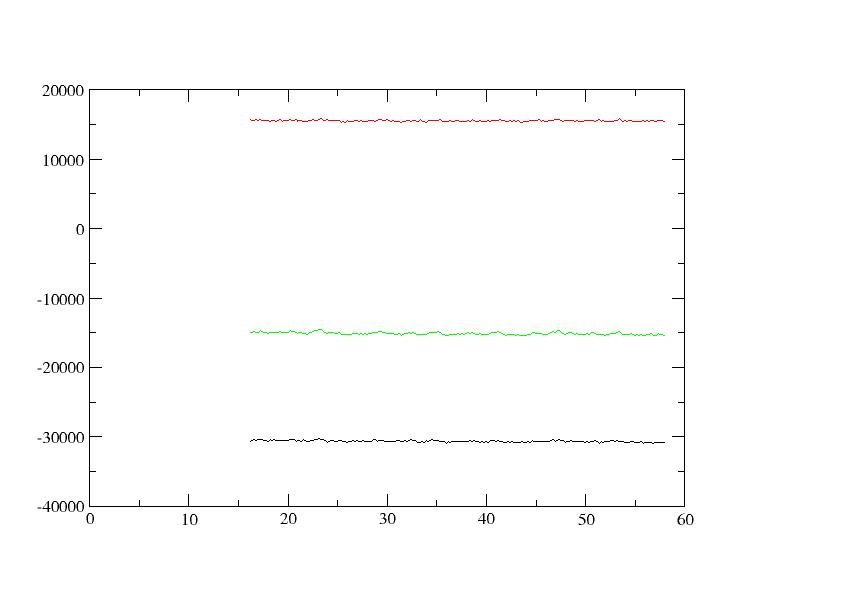# AMBER: Chloride anion ligands leave the protein during MD

From: Francesco Pietra <chiendarret.gmail.com>
Date: Thu, 13 Nov 2008 16:06:06 +0100

Hi:
I have carried out docking of a small protein against a large one, the
latter generated with Modeller from an extremely similar natural
protein and carrying chloride anion ligands. These were accounted for
during docking, and in fact they conserved their position after
docking. The docking region does not involve the chloride anions
directly.

With Amber 10 and pmemd, the complex was first minimized under GB conditions:

&cntrl
imin=1,
maxcyc=3000,
ncyc=1500,
ntb=0,
igb=5,
cut=999
/

Then it was heated gradually to 300K and further equilibrated as follows::

&cntrl
imin=0, irest=0, ntx=1, ntb=0,
igb=5, ntc=2, ntf=2,
ntt=3, gamma_ln=2.0,
nstlim=2500, dt=0.001,
ntpr=100, ntwx=100,
tempi=100.0, temp0=150.0,
cut=999.0, rgbmax=999.0,
nmropt=1
/
&wt TYPE='TEMP0', istep1=0, istep2=2500,
value1=100.0, value2=150.0,
/
&wt TYPE='END'
/

&cntrl
imin=0, irest=0, ntx=1, ntb=0,
igb=5, ntc=2, ntf=2,
ntt=3, gamma_ln=2.0,
nstlim=2500, dt=0.001,
ntpr=100, ntwx=100,
tempi=150.0, temp0=200.0,
cut=999.0, rgbmax=999.0,
nmropt=1
/
&wt TYPE='TEMP0', istep1=0, istep2=2500,
value1=150.0, value2=200.0,
/
&wt TYPE='END'
/

&cntrl
imin=0, irest=0, ntx=1, ntb=0,
igb=5, ntc=2, ntf=2,
ntt=3, gamma_ln=2.0,
nstlim=2500, dt=0.001,
ntpr=100, ntwx=100,
tempi=200.0, temp0=250.0,
cut=999.0, rgbmax=999.0,
nmropt=1
/
&wt TYPE='TEMP0', istep1=0, istep2=2500,
value1=200.0, value2=250.0,
/
&wt TYPE='END'
/

&cntrl
imin=0, irest=0, ntx=1, ntb=0,
igb=5, ntc=2, ntf=2,
ntt=3, gamma_ln=2.0,
nstlim=2500, dt=0.001,
ntpr=100, ntwx=100,
tempi=250.0, temp0=300.0,
cut=999.0, rgbmax=999.0,
nmropt=1
/
&wt TYPE='TEMP0', istep1=0, istep2=2500,
value1=250.0, value2=300.0,
/
&wt TYPE='END'
/

Then increased dt!!

&cntrl
imin=0, irest=0, ntx=1, ntb=0,
igb=5, ntc=2, ntf=2,
ntt=3, gamma_ln=2.0,
nstlim=3000, dt=0.002,
ntpr=100, ntwx=100,
tempi=290.0, temp0=300.0,
cut=999.0, rgbmax=999.0,
nmropt=1
/
&wt TYPE='TEMP0', istep1=0, istep2=600,
value1=290.0, value2=300.0,
/
&wt TYPE='END'
/

As EPTOT ETOT EKTOT were very nearly constant and the whole complex
had not suffered visible distotion, I tried MD of this huge,
unabridged complex under GB conditions in very small chunks as

Production under GB conditions
&cntrl
imin=0, irest=1, ntx=5, ntb=0,
igb=5, ntc=2, ntf=2,
ntt=3, gamma_ln=2.0,
nstlim=3000, dt=0.002,
ntpr=100, ntwx=100,
tempi=300.0, temp0=300.0,
cut=999.0, rgbmax=999.0
/

Starting from production 2, I added nrespa=2 (reaching the guard limit
of 4femtosec)

Surprisingly (for me) the chloride anions started to leave the
protein, while no other distortion showed up. While at frame 0 the
chloride anions are their positions, at frame 50(a base some 25 ps of
trajectory) they are out of the proteins a gradually they disappear
from the screen. Evaluating rmsd with ref frame 0, rmsd increases
linearly with frame number, and in the attached plot. The minimum
potential energy remains at frame 100, out of 250 frames, and ETOT
(black), EPTOT (green), and EKTOT (red) seem OK as in the attached
plot. Nonetheless, might the large nrespa=2 be responsible for the
instability of chloride anion ligands? The size of the system is such
that the 50% gain with nrespa=2 is difficult to renounce to.

Chloride anion was set as Cl- and xleap did not complain about.

Sorry if I made a long story for something trivial, obvious to
experienced people.

Thanks for any kind of comment
francesco pietra

-----------------------------------------------------------------------
The AMBER Mail Reflector
To post, send mail to amber.scripps.edu
To unsubscribe, send "unsubscribe amber" (in the *body* of the email)
to majordomo.scripps.edu(image/jpeg attachment: rmsd_vs0_prod1-8.jpg)(image/jpeg attachment: summary_energies_prod1-8.jpg)

Received on Fri Dec 05 2008 - 14:39:51 PST
Custom Search